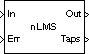Compute filter estimates for input using LMS adaptive filter algorithm

Library

dspobslib

•Description

Note

The LMS Adaptive Filter block is still supported but is likely to be obsoleted in a future release. We strongly recommend replacing this block with the LMS Filter block.

The LMS Adaptive Filter block implements an adaptive FIR filter using the stochastic gradient algorithm known as the normalized least mean-square (LMS) algorithm.

$\begin{array}{c}y\left(n\right)={\stackrel{^}{w}}^{H}\left(n-1\right)u\left(n\right)\\ e\left(n\right)=d\left(n\right)-y\left(n\right)\\ \stackrel{^}{w}\left(n\right)=\stackrel{^}{w}\left(n-1\right)+\frac{u\left(n\right)}{a+{u}^{H}\left(n\right)u\left(n\right)}\mu {e}^{*}\left(n\right)\end{array}$

The variables are as follows.

VariableDescription

n

The current algorithm iteration

u(n)

The buffered input samples at step n

$\stackrel{^}{w}\left(n\right)$

The vector of filter-tap estimates at step n

y(n)

The filtered output at step n

e(n)

The estimation error at step n

d(n)

The desired response at step n

µ

To overcome potential numerical instability in the tap-weight update, a small positive constant (a = 1e-10) has been added in the denominator.

To turn off normalization, clear the Use normalization check box in the parameter dialog. The block then computes the filter-tap estimate as

$\stackrel{^}{w}\left(n\right)=\stackrel{^}{w}\left(n-1\right)+u\left(n\right)\mu {e}^{*}\left(n\right)$

The block icon has port labels corresponding to the inputs and outputs of the LMS algorithm. Note that inputs to the In and Err ports must be sample-based scalars. The signal at the Out port is a scalar, while the signal at the Taps port is a sample-based vector.

Block PortsCorresponding Variables

In

u, the scalar input, which is internally buffered into the vector u(n)

Out

y(n), the filtered scalar output

Err

e(n), the scalar estimation error

Taps

$\stackrel{^}{w}\left(n\right)$, the vector of filter-tap estimates

An optional Adapt input port is added when you select the Adapt input check box in the dialog. When this port is enabled, the block continuously adapts the filter coefficients while the Adapt input is nonzero. A zero-valued input to the Adapt port causes the block to stop adapting, and to hold the filter coefficients at their current values until the next nonzero Adapt input.

The FIR filter length parameter specifies the length of the filter that the LMS algorithm estimates. The Step size parameter corresponds to µ in the equations. Typically, for convergence in the mean square, µ must be greater than 0 and less than 2. The Initial value of filter taps specifies the initial value $\stackrel{^}{w}\left(0\right)$ as a vector, or as a scalar to be repeated for all vector elements. The Leakage factor specifies the value of the leakage factor, 1 –μ α, in the leaky LMS algorithm below. This parameter must be between 0 and 1.

$\stackrel{^}{w}\left(n+1\right)=\left(1-\mu \alpha \right)\stackrel{^}{w}\left(n\right)+\frac{u\left(n\right)}{{u}^{H}\left(n\right)u\left(n\right)}\mu {e}^{*}\left(n\right)$

Parameters

FIR filter length

The length of the FIR filter.

Step-size

The step-size, usually in the range (0, 2). Tunable (Simulink).

Initial value of filter taps

The initial FIR filter coefficients.

Leakage factor

The leakage factor, in the range [0, 1]. Tunable (Simulink).

Use normalization

Select this check box to compute the filter-tap estimate using the normalized equations.

Enables the Adapt port when selected.

References

Haykin, S. Adaptive Filter Theory. 3rd ed. Englewood Cliffs, NJ: Prentice Hall, 1996.

Supported Data Types

• Double-precision floating point

• Single-precision floating point

 Kalman Adaptive Filter (Obsolete) DSP System Toolbox RLS Adaptive Filter (Obsolete) DSP System Toolbox

See Noise Cancellation in Simulink Using Normalized LMS Adaptive Filter for related information.

Introduced in R2008b

DSP System Toolbox DocumentationGet trial now